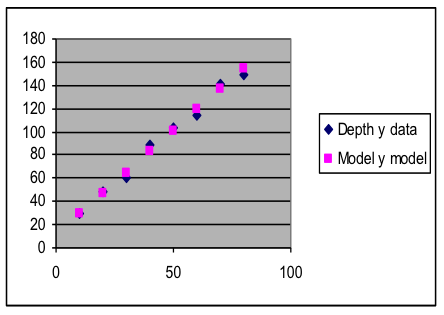## I1.05: Section 3 Part 2

### Example 3: Using Models.xls to fit a linear model to a dataset

The table to the right gives data on the measured rate at which sediment built up in a factory hold­ing tank during routine operation, after a cleaning process that is repeated a couple of times per year. The factory operators want to use this information to make a formula to predict sediment depth at any chosen time after a cleaning.

A preliminary graph of the data shows that the pattern of the points is reasonably close to a straight line. Therefore, the “Linear Model” worksheet in Models.xls is the appropriate one to use.

 Days since cleaning Depth (mm) 10 29.9 20 48.0 30 60.5 40 88.6 50 102.9 60 114.1 70 141.1 80 149.5

Solution:

In an earlier topic, you used a spreadsheet to adjust the intercept and slope of a linear equation and saw the resulting changes in the position of the straight-line graph. We will now use that same technique to make a good linear model for this data with Models.xls.

1. Insert a new worksheet into Models.xls, labeling its tab “Linear Sediment Model”. Then copy into the new worksheet the contents of the read-only worksheet labeled “Linear Model Template”.
2. In this case we want to predict sediment depth for any given number of days since the last cleaning. This means that we want to use day as the input variable x and depth as the output variable y.
3. Copy the data to the spreadsheet (columns A and B, rows 3 to 10 for the numbers), then label the top of the data columns with “Days” in A2 and “Depth” in B2.
4. Select C3 (which contains a preset linear formula based on the values in G3 and G4) and spread the formula down to row 10, matching the data. At first, these model values will be zeros.
5. Also select and spread D3 and E3 down to row 10. The values in columns D and E will not be very meaningful until you adjust the model to be a good fit.
6. Make a scatter plot of the data and model columns together (that is, the rectangle A1:C10). At first, the model points will lie on a horizontal line along the x-axis.
7. Adjust the parameters in G3 and G4 so that the model points are as close as you can get them to the data points.

For a linear model, here is a good parameter-adjustment strategy:

1. Set the intercept to approximately where the data trend crosses the y axis (about 10 in this case, although you do not need to be exactly right since you will adjust the intercept again in [c] below),
2. Adjust the slope to make the model line parallel to the data trend (in this case, 1 is too low a value for the slope, and 2 is too high; 1.8 seems about right).
3. Now adjust the intercept to its best value, moving the model line without changing its slope until the model goes right through the data (in this case, a value of 11 for the intercept works well).
1. Check to see if the model is good. In this case, the model points are close to the data points over the whole data range, showing that a linear model is the correct type to use for this data.
2. Write the mathematical formula for the model you have found: y = 1.8 x + 11

The Linear Sediment Model worksheet should look about like this at the end of this solution process:

 A B C D E F G 1 x y data y model Data-Model Linear model: y = m * x + b 2 Days Depth Prediction deviation y = 1.8x+11 3 10 29.9 29 0.9 11 b: Intercept 4 20 48.0 47 1 1.8 m: Slope 5 30 60.5 65 -4.5 6 40 88.6 83 5.6 7 50 102.9 101 1.9 8 60 114.1 119 -4.9 9 70 141.1 137 4.1 10 80 149.5 155 -5.5 11 12 13